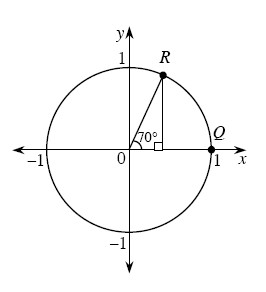### Home > A2C > Chapter 8 > Lesson 8.1.4 > Problem8-65

8-65.

A $70°$ angle is drawn for you in the unit circle below.

1. Approximate the coordinates of point R.

$\left(0.3420, 0.9397\right)$

2. How could you represent the exact coordinates of point R?

$\left(\operatorname{cos}70°, \operatorname{sin}70°\right)$